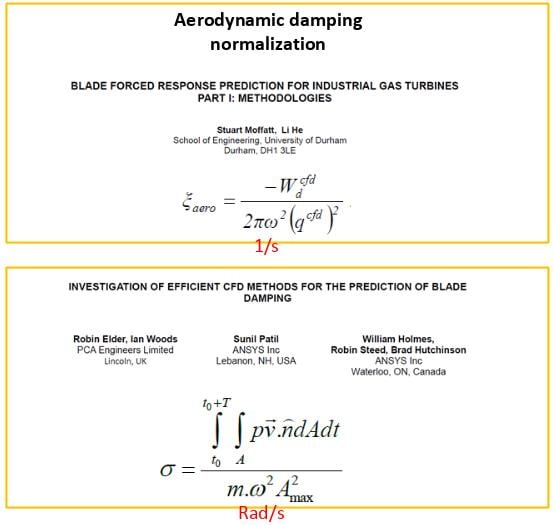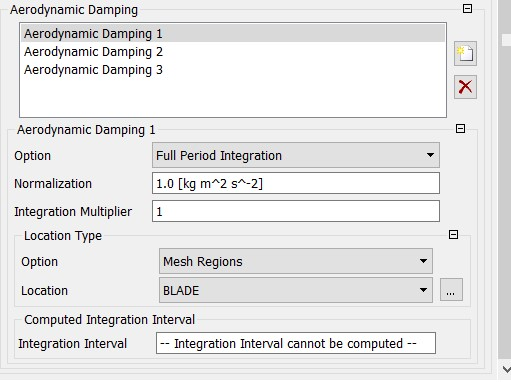## Fluids

•Tayyip
Subscriber

I can calculate aerodynamic damping as a function of ib phase angle in CFX for imported modeshapes. Those are work done by the fluid on the blades in units of Joule per vibration cycle. I have trouble in proper normalization of this damping for ANSYS forced response analyses. In the attached picture file, there are snapshots from 2 publications. There are two different normalizations, which are applied to ANSYS aeromechanics (harmonic balance analysis for forced response). The difference is actually a factor of  "2*pi" as one defines mode frequency as rad/sec, and another defines it as 1/s. Which one is true for the specific forced response analyses implemented in ANSYS?•Bill Holmes
Ansys Employee

Hi Tayyip,

I think these two equations are showing something slightly different.  In the Durham paper, they are computing a (critical) aerodynamic damping ratio and in the PCA/ANSYS paper they are computing an aerodynamic damping coefficient.

We typically don't recommend using the work per cycle or aero damping directly for forced response calculations, since you will lose the stiffness component.  You would be best off to follow the procedures shown in this section of the help documentation:

https://ansyshelp.ansys.com/account/secured?returnurl=/Views/Secured/corp/v191/ans_cycsym/cycsym_aero_coupling.html

But if that caveat does not scare you, then you could use work per cycle.  In that case what ANSYS is going to need for the forced response calculation is the modal force.  The relationship for this as shown in the help documentation is

Modal Force = -Wcyc/(PI*scaling)

There is no 2 with the PI, as it is cancelled out in the calculations between Wcyc, critical damping, and modal force.

The scaling is essentially undoing any scaling that happened between structural and CFD.

Regards,

Bill Holmes

•Tayyip
Subscriber

Hi Bill,

Thank you so much for your help.

With Regards,

Tayyip

•Jiqing
Subscriber

Hi Bill,

I want to know how to set the normalized value in CFX to calculate the aerodynamic damping. As shown in the picture attached below, the value set in Normalization is used to normalize the aerodynamic work per cycle. According to the formula shown in the first picture attached by Tayyip, this value should be the denominator of the formula. So how to calculate value? Is it based the Modal Analysis?Best Regards,

Jiqing Cong